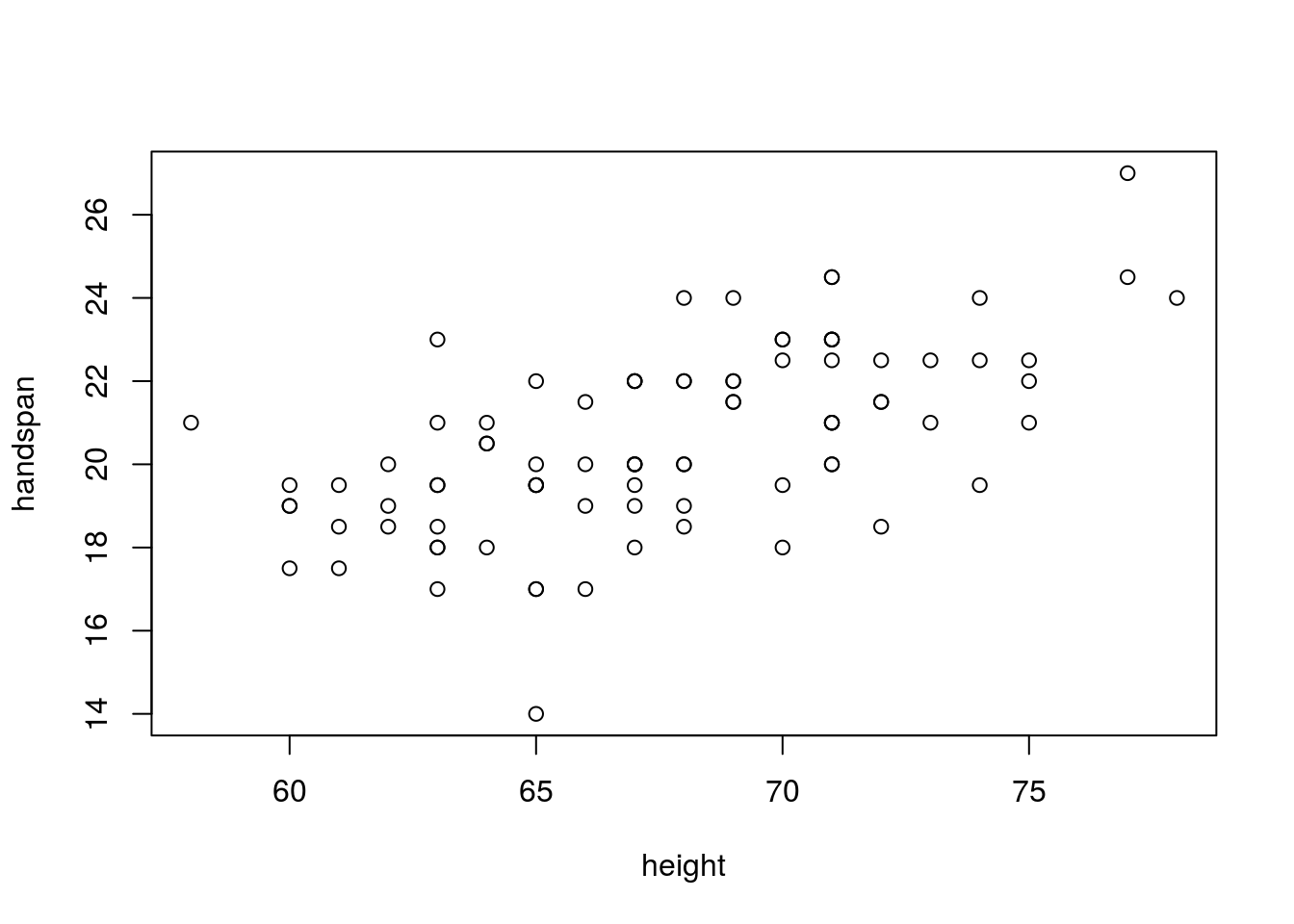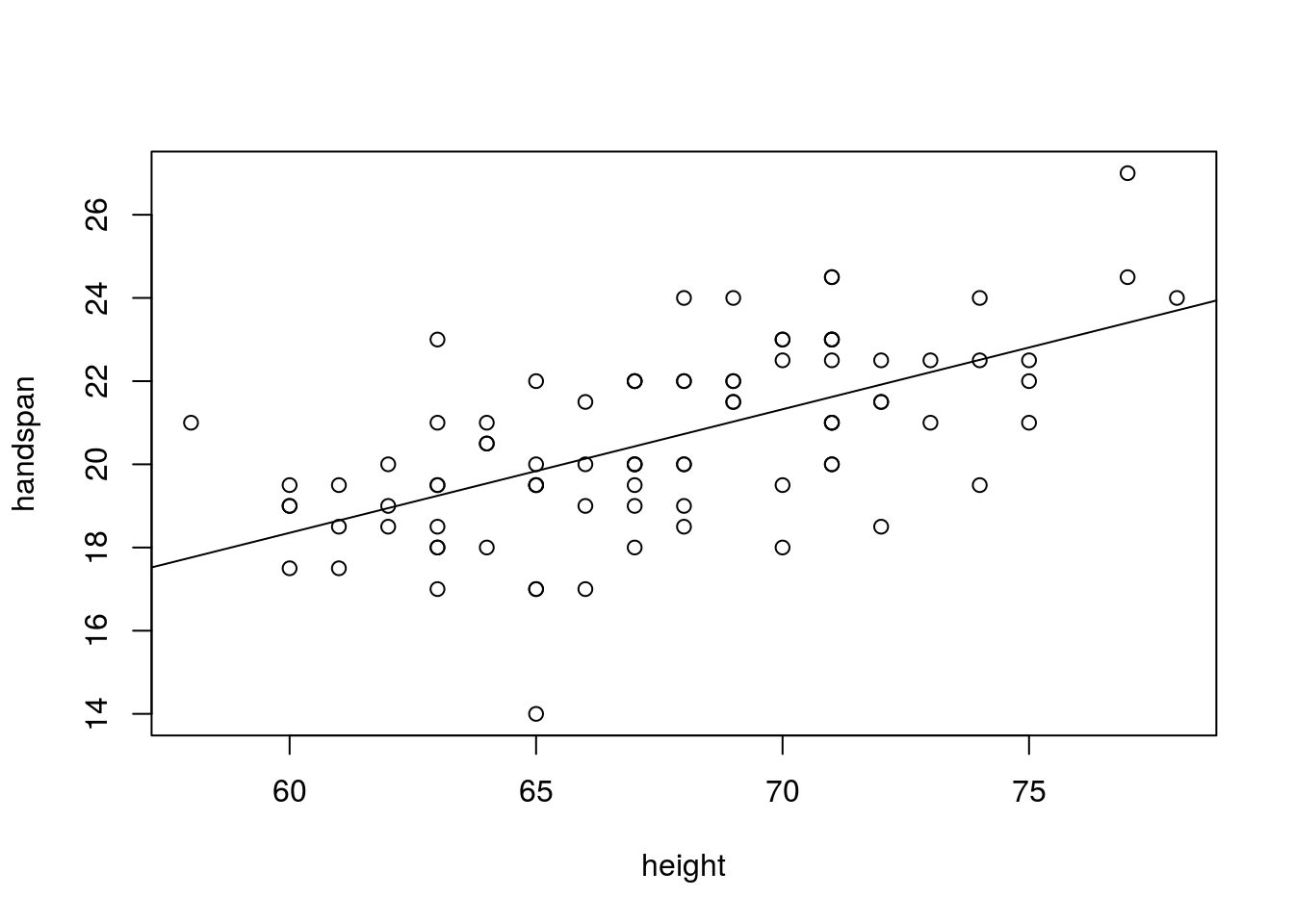This tutorial has two parts. The first part shows you how to calculate the remaining summary statistics from the lectures and introduces a few new plotting commands. The second part describes one of the most fundamental parts of R: creating your own functions. Rather than appearing at the end, several exercises are scattered throughout the parts of this tutorial.

## More Summary Statistics

First we’ll load the survey data from R Tutorial #2.

Like before we’ll only need a subset of the columns available; rather than go to the hassle of deleting these columns afterwards, we can specify which columns to keep simultaneously with reading in the data using the `select` argument to `fread`. (There is also a `drop` argument for the reverse – specifying columns to exclude)

``````library(data.table)
select = c("handedness", "height", "handspan"))``````

### Correlation: `cor`

This command calculates sample correlation coefficients. If you pass it two vectors of the same length it returns the correlation between them. If you feed it a `data.table` or `matrix` it returns a matrix containing all pairwise correlations between the columns (we haven’t covered matrices in R since you’ll only need to interpret them as output for this class – for a primer on handling `matrix` objects, see here). Unlike the univariate summary statistics, in which we used `na.rm = TRUE` to ignore missing observations, for `cor` we use the argument `use = "complete.obs"`. The reason for this difference is that `cor` can handle a `matrix` or `data.table` as its input. In this case there are actually many different ways of handling missing observations. For more details, see `?cor`. Setting `use = "complete.obs"` removes any rows with missing observations before proceeding.

``survey[ , cor(handspan, height, use = "complete.obs")]``
``##  0.6042423``

Of course, we could use the approach we saw in R Tutorial #2 as well; whichever feels more natural:

``survey[!is.na(handspan) & !is.na(height), cor(handspan, height)]``
``##  0.6042423``

We can also feed the full `data.table` to `cor` like so:

``cor(survey, use = "complete.obs")``
``````##             handedness    height    handspan
## handedness  1.00000000 0.1125605 -0.01719909
## height      0.11256055 1.0000000  0.60942759
## handspan   -0.01719909 0.6094276  1.00000000``````
``````#alternatively, there's the na.omit
#  function, which does just what it says:
cor(na.omit(survey))``````
``````##             handedness    height    handspan
## handedness  1.00000000 0.1125605 -0.01719909
## height      0.11256055 1.0000000  0.60942759
## handspan   -0.01719909 0.6094276  1.00000000``````

We see that there is a large positive correlation between `height` and `handspan` and a slight positive correlation between `height` and `handedness.` There’s basically no correlation between `handedness` and `handspan`. The preceding matrix has ones on its main diagonal since the correlation between any variable and itself is identically one. (A good exercise for extra practice would be to prove this assertion using the formula for correlation from class. It’s not very difficult.)

If you look carefully, you’ll notice that the correlation between `height` and `handspan` is slightly different when calculated at the same time as all the other correlations. This is because of the way that `use = "complete.obs"` drops rows. In this case, it indicates that there is at least one observation in our dataset for which we have `height` and `handspan` but not `handedness`.

For practice, we can find this with judicious use of `is.na` and logical operators:

``survey[is.na(handedness) & !is.na(height) & !is.na(handspan)]``
``````##    handedness height handspan
## 1:         NA     70     19.5``````

### Covariance: `cov`

This command works just like `cor` but returns covariances rather than correlations:

``survey[ , cov(handspan, height, use = "complete.obs")]``
``##  5.910786``
``cov(survey, use = "complete.obs")``
``````##            handedness    height   handspan
## handedness  0.1685042  0.206959 -0.0155499
## height      0.2069590 20.062517  6.0121751
## handspan   -0.0155499  6.012175  4.8510260``````
``cov(na.omit(survey))``
``````##            handedness    height   handspan
## handedness  0.1685042  0.206959 -0.0155499
## height      0.2069590 20.062517  6.0121751
## handspan   -0.0155499  6.012175  4.8510260``````

### Regression: `lm` and `abline`

This command stands for “_l_inear _m_odel" and is R’s general-purpose regression command. Its syntax is similar to that of `boxplot` from R Tutorial #2 in that we use a tilde (`~`) to indicate a functional relationship. Here the variable to the left of the tilde is the “y” variable, while the variable to the right is the “x” variable.

``survey[ , lm(height ~ handspan)]``
``````##
## Call:
## lm(formula = height ~ handspan)
##
## Coefficients:
## (Intercept)     handspan
##      42.251        1.229``````

Remember: unlike correlation and covariance, regression is not symmetric:

``survey[ , lm(handspan ~ height)]``
``````##
## Call:
## lm(formula = handspan ~ height)
##
## Coefficients:
## (Intercept)       height
##      0.5305       0.2970``````

It turns out that we can use the same syntax with the command `plot`:

``survey[ , plot(handspan ~ height)]``To add the regression line, we follow the `plot` command with the function `abline` like so:

``````survey[ , plot(handspan ~ height)]
survey[ , abline(lm(handspan ~ height))]``````Note that `abline` can only be used after you’ve already made a plot. It adds a line to the existing plot rather than making a plot of its own.

You can also use `abline` to plot different kinds of lines. For example, we can show that the regression line goes through the means of the data as follows:

``````survey[ , {
plot(handspan ~ height)
abline(lm(handspan ~ height))
abline(v = mean(height, na.rm = TRUE),
h = mean(handspan, na.rm = TRUE),
col = 'red', lty = 2)
}]``````